# 2.4 Heterogeneous Track Pairing (HTP)

## 2.4 Heterogeneous Track Pairing (HTP)

We describe this technique in two steps. First, we describe how it works for a single multi-zone disk drive. Next, we extend the discussion to a heterogeneous collection of disk drive. Finally, we discuss the tradeoff associated with this technique.

Assuming a single disk drive (say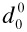) with #TR0 tracks, Track Pairing  pairs the innermost track (TR#TR0 - 1()) with the outermost track (TR0()), working itself towards the center of the disk drive. The result is a logical disk drive that consists of #TR0/2 logical tracks that have approximately the same storage capacity and transfer rate. This is based on the (realistic) assumption that the storage capacity of tracks increases linearly as one moves from the innermost track to the outermost track. Using this logical disk drive, the system may utilize one of the traditional continuous display techniques in support of hiccup-free display.

Assuming a heterogeneous configuration consisting of K disk models, HTP utilizes Track Pairing to construct track pairs for each disk. If the number of disks for each disk model is identical (q0= q1== qK-1), HTP constructs qi groups of disk drives consisting of one disk from each of the K disk models. Next, it realize a logical track that consists of K track pairs, one track pair from each disk drive in the group. These logical tracks constitute a logical disk. Obviously, the disk with the fewest number of tracks determines the total number of logical tracks for each logical disk. With such a collection of homogeneous logical disks, one can use one of the popular hiccup-free display techniques. For example, similar to both OLT1 and OLT2, one can stripe a video clip into blocks and assign the blocks to the logical disks in a round-robin manner.

HTP wastes disk space in two ways. First, the number of tracks in a logical disk is determined by the physical disk drive with fewest track pairs. For example, if a configuration consists of two heterogeneous disks, one with 20,000 track pairs and the other with 15,000 track pairs, then the resulting logical disk will consist of 15,000 track pairs. In essence, this technique eliminates 5,000 track pairs from the first disk drive. Second, while it is realistic to assume that the storage capacity of each track increases linearly from the innermost track to the outermost one, it is not 100% accurate . Once the logical tracks are realized, the storage capacity of each logical track is determined by the track with the lowest storage capacity.

### 2.5 Heterogeneous FIXB

In order to describe this technique, we first describe how the system guarantees continuous display with a single multi-zone disk drive. Next, we describe the extensions of this technique to a heterogeneous disk drive.

#### 2.5.1 FIXB with One Multi-zone Disk 

With this technique, the blocks of an object X are rendered equi-sized. Let B denote the size of a block. The system assigns the blocks of X to the zones in a round-robin manner starting with an arbitrary zone. FIXB configures the system to support a fixed number of simultaneous displays, N. This is achieved by requiring the system to scan the disk in one direction, say starting with the outermost zone moving inward, visiting one zone at a time and multiplexing the bandwidth of that zone among N block reads. Once the disk arm reads N blocks from the innermost zone, it is repositioned to the outermost zone to start another sweep of the zones. The time to perform one such sweep is denoted as Tscan. The system is configured to produce and display an identical amount of data per Tscan period. The time required to read N blocks from zone i, denoted TMUX(Zi), is dependent on the transfer rate of zone i. This is because the time to read a block (Tdisk(Zi)) during one TMUX(Zi) is a function of the transfer rate of a zone.

During each TMUX period, N active displays might reference different objects. This would force the disk to incur a seek when switching from the reading of one block to another, termed TW_Seek. TW_seek also includes the rotational latency. At the end of a Tscan period, the system observes a long seek time (Tcseek) attributed to the disk repositioning its arm to the outermost zone. The disk produces m blocks of X during one Tscan period (m B bytes). The number of bytes required to guarantee a hiccup-free display of X during Tscan should either be lower than or equal to the number of bytes produced by the disk. This constraint is formally stated as:

 (35.2)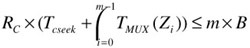The amount of memory required to support a display is minimized when the left hand side of Eq. 35.2 equals its right hand side.

During a TMUX, N blocks are retrieved from a single zone, ZActive. In the next TMUX period, the system references the next zone Z(Active+1) mod m. When a display references object X, the system computes the zone containing X0, say Zi. The transfer of data on behalf of X does not start until the active zone reaches Zi. One block of X is transferred into memory per TMUX. Thus, the retrieval of X requires f such periods. (The display of X may exceed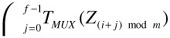seconds as described below.) The memory requirement for displaying object X varies due to the variable transfer rate. This is best illustrated using an example. Assume that the blocks of X are assigned to the zones starting with the outermost zone, Z0. If ZActive is Z0 then this request employs one of the idle Tdisk(Z0) slots to read X0. Moreover, its display can start immediately because the outermost zone has the highest transfer rate. The block size and N are chosen such that the data accumulates in memory when accessing outermost zones and decreases when reading data from innermost zones on behalf of a display. In essence, the system uses buffers to compensate for the low transfer rates of innermost zones using the high transfer rates of outermost zones, harnessing the average transfer rate of the disk. Note that the amount of required memory reduces to zero at the end of one Tscan in preparation for another sweep of the zones.

The display of an object may not start upon the retrieval of its block from the disk drive. This is because the assignment of the first block of an object may start with an arbitrary zone while the transfer and display of data is synchronized relative to the outermost zone, Z0. In particular, if the assignment of X0 starts with a zone other than the outermost zone (say Zi, i 0) then its display might be delayed to avoid hiccups. The duration of this delay depends on: 1) the time elapsed from retrieval of X0 to the time that block Xm-1 is retrieved from zone Z0, termed TaccessZ0, and 2) the amount of data retrieved during TaccessZ0. If the display time of data corresponding to item 2 (Tdisplay(m-i)) is lower than TaccessZ0, then the display must be delayed by TaccessZ0 - Tdisplay(m-i). To illustrate, assume that X0 is assigned to the innermost zone Zm-1 (i.e., i=m-1) and the display time of each of its block is 4.5 seconds, i.e., Tdisplay(1)=4.5 seconds. If 10 seconds elapse from the time X0 is read until X1 is read from Z0 then the display of X must be delayed by 5.5 seconds relative to its retrieval from Zm-1. If its display is initiated upon retrieval, it may suffer from a 5.5 second hiccup. This delay to avoid a hiccup is shorter than the duration of a Tscan. Indeed, the maximum latency observed by a request is Tscan when the number of active displays is less than N.

 (35.3)This is because at most N displays might be active when a new request arrives referencing object X. In the worst case scenario, these requests might be retrieving data from the zone that contains X0 (say Zi) and the new request arrives too late to employ the available idle slot. (Note that the display may not employ the idle slot in the next TMUX because Zi+1 is now active and it contains X1 instead of X0.) Thus, the display of X must wait one Tscan period until Zi becomes active again.

TMUX(Zi) can be defined as:

 (35.4)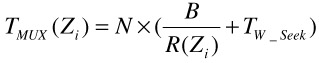Substituting this into Eq. 35.2, the block size is defined as:

 (35.5)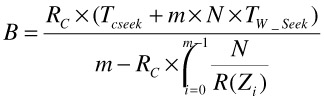Observe that FIXB wastes disk space when the storage capacity of the zones is different. This is because once the storage capacity of the smallest zone is exhausted then no additional objects can be stored as they would violate a round-robin assignment.

#### 2.5.2 Extensions of FIXB (HetFIXB)

With a heterogeneous collection of disks, we continue to maintain a Tscan per disk drive. While the duration of a Tscan is identical for all disk drives, the amount of data produced by each Tscan is different. We compute the block size for each disk model (recall that blocks are equi-sized for all zones of a disk) such that the faster disks compensate for the slower disks by producing more data during their Tscan period. HetFIXB aligns the Tscan of each individual disk drive with one another such that they all start and end in a Tscan.

To support N simultaneous displays, HetFIXB must satisfy the following equations.

 (35.6)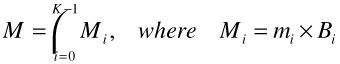(35.7)(35.8)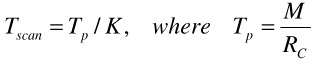(35.9)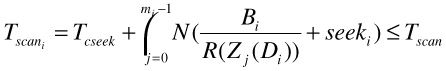where 0 i < K.

To illustrate, assume a configuration consisting of 3 disks; see Figure 35.4. Assume the average transfer rates of disks, AvgR0 = 80 Mbps, AvgR1 = 70 Mbps, and AvgR2 = 60 Mbps, respectively. When Rc = 4 Mbps, 1.5 Mbytes of data (M = 1.5 MB) is required every 3 seconds (TP = 3 sec) to support a hiccup-free display. Based on the ratio among the average transfer rates of disk models, M0 = 0.5715 MB, M1 = 0.5 MB, and M2 = 0.4285 MB. Thus, B0 = M0 / m0 = 0.19 MB, B1 = M1 / m1 = 0.25 MB, B2 = M2 / m2 = 0.14 MB. An object X is partitioned into blocks and blocks are assigned into zones in a round-robin manner. When a request for X arrives, the system retrieves X0, X1, and X2 (M0 = 3 B0 amount of data) from D0 during the first Tscan. A third of M (0.5 MB) is consumed during the same Tscan. Hence, some amount of data, 0.0715 MB, remains un-consumed in the buffer. In the next Tscan, the system retrieves X3 and X4 (M1 = 2 B1 amount of data) from D1. While the same amount of data (0.5 MB) is retrieved and consumed during this Tscan, the accumulated data (0.0715 MB) still remains in the buffer. Finally, during the last Tscan, the system retrieves X5, X6, and X7 (M2 = 3 B2 amount of data) from D2. Even though the amount of data retrieved in this Tscan (0.4285 MB) is smaller than the amount of data displayed during a Tscan (0.5 MB), there is no starvation because 0.0715 megabytes of data is available in the buffer. This process is repeated until the end of display.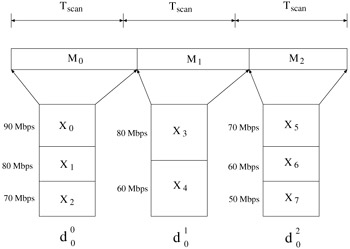Figure 35.4: HetFIXB.

### 2.6 Heterogeneous Deadline Driven (HDD)

With this technique, blocks are assigned across disk drives in a random manner. A client issues block requests, each tagged with a deadline. Each disk services block requests with the Earliest Deadline First policy. In , we showed that the assignment of blocks to the zones in a disk should be independent of the frequency of access to the blocks. Thus, blocks are assigned to the zones in a random manner. The size of the blocks assigned to each disk model is different. They are determined based on the average weighted transfer rate of each disk model. Let WRi denote the weighted average transfer rate of disk model i:

 (35.10)(35.11)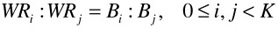Assuming Bi Bj where i < j and 0 i,j < K, an object X is divided into blocks such that the size of each block Xi is Bi mod k. Blocks with the size of Bi are randomly assigned to disks belonging to model i. A random placement may incur hiccups that are attributed to the statistical variation of the number of block requests per disk drive, resulting in varying block retrieval time. Traditionally, double buffering has been widely used to absorb the variance of block retrieval time: while a block in a buffer is being consumed, the system fills up another buffer with data. However, we generalize double buffering to N buffering and prefetching N-1 buffers before initiating a display. This minimizes the hiccup probability by absorbing a wider variance of block retrieval time, because data retrieval is N-1 blocks ahead of data consumption.

We assume that, upon a request for a video clip X, a client: (1) concurrently issues requests for the first N-1 blocks of X (to prefetch data), (2) tags the first N-1 block requests, Xi (0 i < N), with a deadline,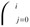, (3) starts display as soon as the first block (X0) arrives. For example, when N=4, first three blocks are requested at the beginning. Then, the next block request is issued immediately after the display is initiated. Obviously, there are other ways of deciding both the deadline of the prefetched blocks and when to initiate display blocks. In , we analyzed the impact of these alternative decisions and demonstrated that the combination of the above two choices enhances system performance.

### 2.7 Disk Grouping (DG)

Assuming a fixed data transfer rate of a disk (single-zone disk model), this technique groups physical disks into logical ones and assumes a uniform characteristic for all logical disks. To illustrate, the six physical disks of Figure 35.5 are grouped to construct two homogeneous logical disks. In this figure, a larger physical disk denotes a newer disk model that provides both a higher storage capacity and a higher performance. The blocks of a movie X are assigned to the logical disks in a round-robin manner to distribute the load of a display evenly across the available resources [2, 23, 8]. A logical block is declustered  across the participating physical disks. Each piece is termed a fragment (e.g., X0.0 in Figure 35.5). The size of each fragment is determined such that the service time (Tservice) of all physical disks is identical.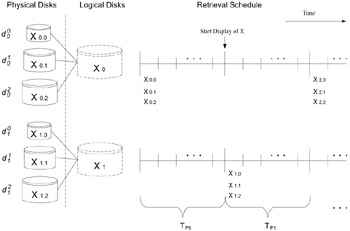Figure 35.5: Disk grouping.

When the system retrieves a logical block into memory on behalf of a client, all physical disks are activated simultaneously to stage their fragments into memory. This block is then transmitted to the client. To guarantee a hiccup-free display, the display time of a logical block (Bl) must be equivalent to the duration of a time period: Tp=Bl/Rc. Moreover, if a logical disk services N simultaneous displays then the duration of each time period must be greater or equal to the service time to read N fragments from every physical disk drive i that is a part of the logical disk. Thus, the following constraint must be satisfied:

 (35.12)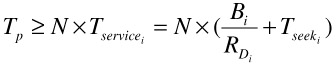Because the time period must be equal for all physical drives (K) that constitutes a logical disk, we obtain the following equations:

 (35.13)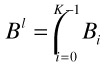(35.14)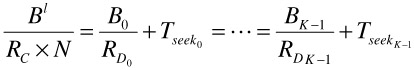To support N displays with one logical disk, the system requires 2 N memory buffers. Thus the total number of displays supported by the system (Ntot) is N the number of logical disks, and the required memory space is 2 Ntot Bl.

### 2.8 Disk Merging (DM)

Using a fixed data transfer rate of a disk as in DG, this technique separates the concept of logical disk from the physical disks all together. Moreover, it forms logical disks from fractions of the available bandwidth and storage capacity of the physical disks; see Figure 35.6. These two concepts are powerful abstractions that enable this technique to utilize an arbitrary mix of physical disk models. The design of this technique is as follows. First, it chooses how many logical disks should be mapped to each of the slowest physical disks and denotes this factor with p0. Note that we can choose any number of logical disks from the slowest physical disk by taking a fraction of disk bandwidth and storage capacity of the disk. For example, in Figure 35.6, the two slowest disks d2 and d3 each represent 1.5 logical disks, i.e., p0=1.5. Then, the time period TP and the block size necessary to support p0 logical disks on a physical disk can be established as follows:

 (35.15)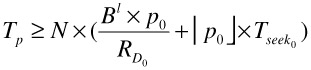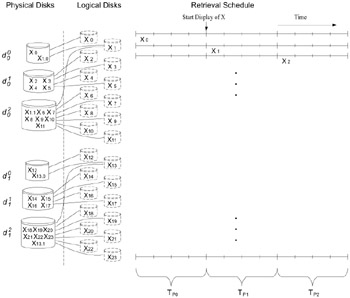Figure 35.6: Disk merging.

 (35.16)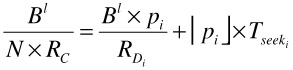Because of the ceiling-function there is no closed formula solution for pi from the above equation. However, numerical solutions can be easily found. An initial estimate for pi may be obtained from the bandwidth ratio of the two different disk types involved. When iteratively refined, this value converges rapidly towards the correct ratio. In the example configuration of Figure 35.6, the physical disks d1 and d4 realize 2.5 logical disks each (p1=2.5) and the disks d0 and d3 support 3.5 logical disks (p2=3.5). Note that fractions of logical disks (e.g., 0.5 of d0 and 0.5 of d3) are combined to form additional logical disks (e.g., ld7, which contains block X7 in Figure 35.6). The total number of logical disks (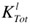) is defined as:

 (35.17)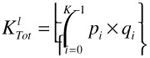Once a homogeneous collection of logical disks is formed, the block size Bl of a logical disk determines the total amount of memory with the total number of simultaneous displays (NTot = N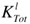); see .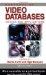Handbook of Video Databases: Design and Applications (Internet and Communications)
ISBN: 084937006X
EAN: 2147483647
Year: 2003
Pages: 393